# A distribution that has been used to model tolerance levels in bioassays is the logisticdistribution with parameters a and ß. The cumulative distribution function of the logisticdistribution isF(x) = [1+e--a)/The parameter a may be any real number; the parameter B may be any positive number. LetX be a random variable with this distribution.Find the probability density function fx(x).a.Show that fx(x) is symmetric around a, that is, fy(« – x) = f;(a +x) forall x.b.Explain why the symmetry described in part (b) shows that µx = a. You may assumeC.that µy exists.

Question
1 views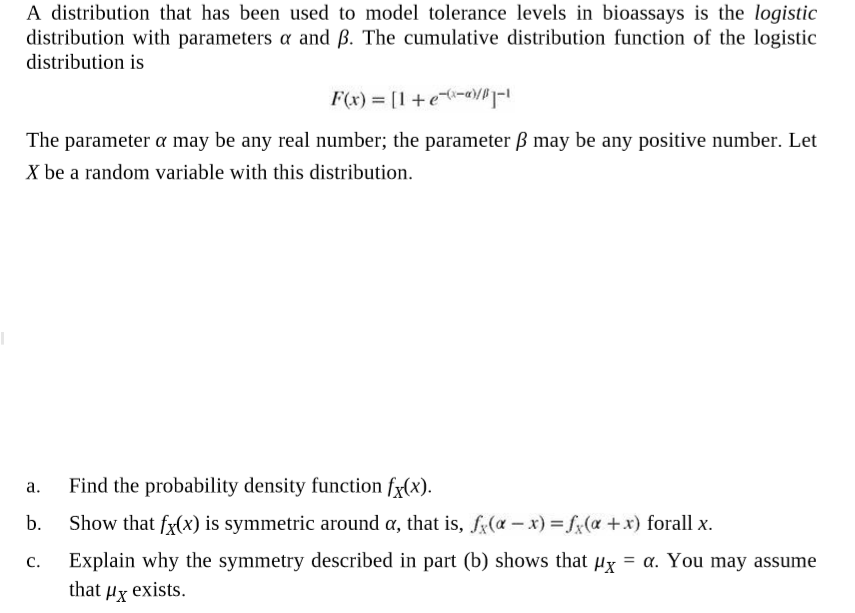help_outlineImage TranscriptioncloseA distribution that has been used to model tolerance levels in bioassays is the logistic distribution with parameters a and ß. The cumulative distribution function of the logistic distribution is F(x) = [1+e--a)/ The parameter a may be any real number; the parameter B may be any positive number. Let X be a random variable with this distribution. Find the probability density function fx(x). a. Show that fx(x) is symmetric around a, that is, fy(« – x) = f;(a +x) forall x. b. Explain why the symmetry described in part (b) shows that µx = a. You may assume C. that µy exists. fullscreen
check_circle

Step 1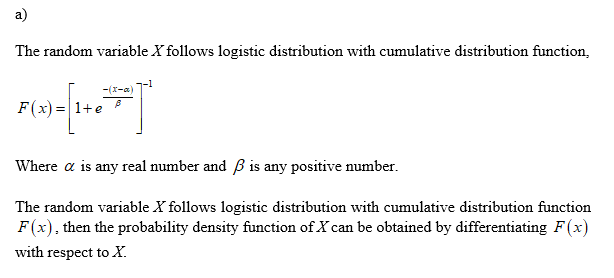Step 2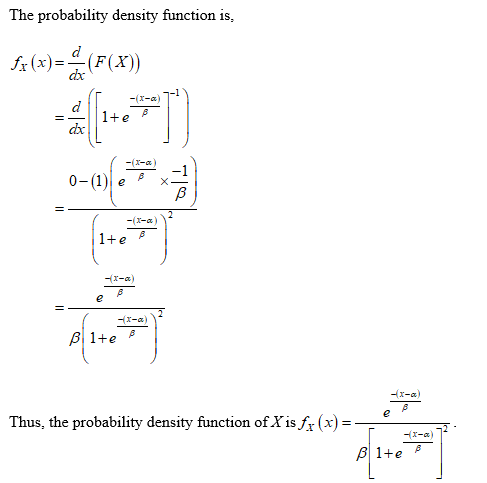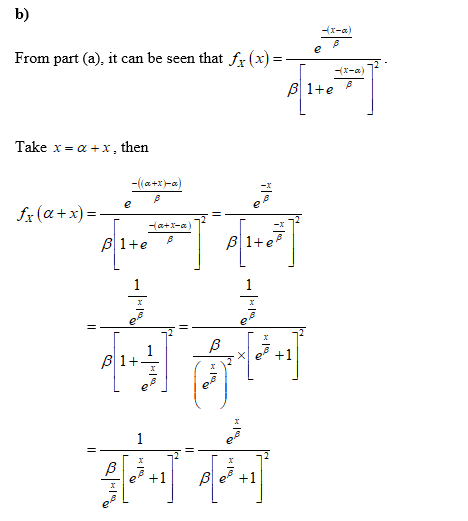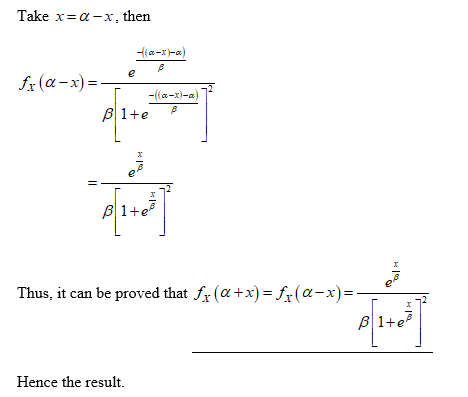...

### Want to see the full answer?

See Solution

#### Want to see this answer and more?

Solutions are written by subject experts who are available 24/7. Questions are typically answered within 1 hour.*

See Solution
*Response times may vary by subject and question.
Tagged in